Question

# Without using the Pythagorean theorem, prove that two right triangles are congruent if the hypotenuse and...

Without using the Pythagorean theorem, prove that two right triangles are congruent if the hypotenuse and leg of one are equal to the hypotenuse and leg of the other.

Do this with placing the triangles so that there equal legs coincide and their right legs are adjacent. This will form a large isoceles triangle. Use this to show that the given triangles are congruent by AAS.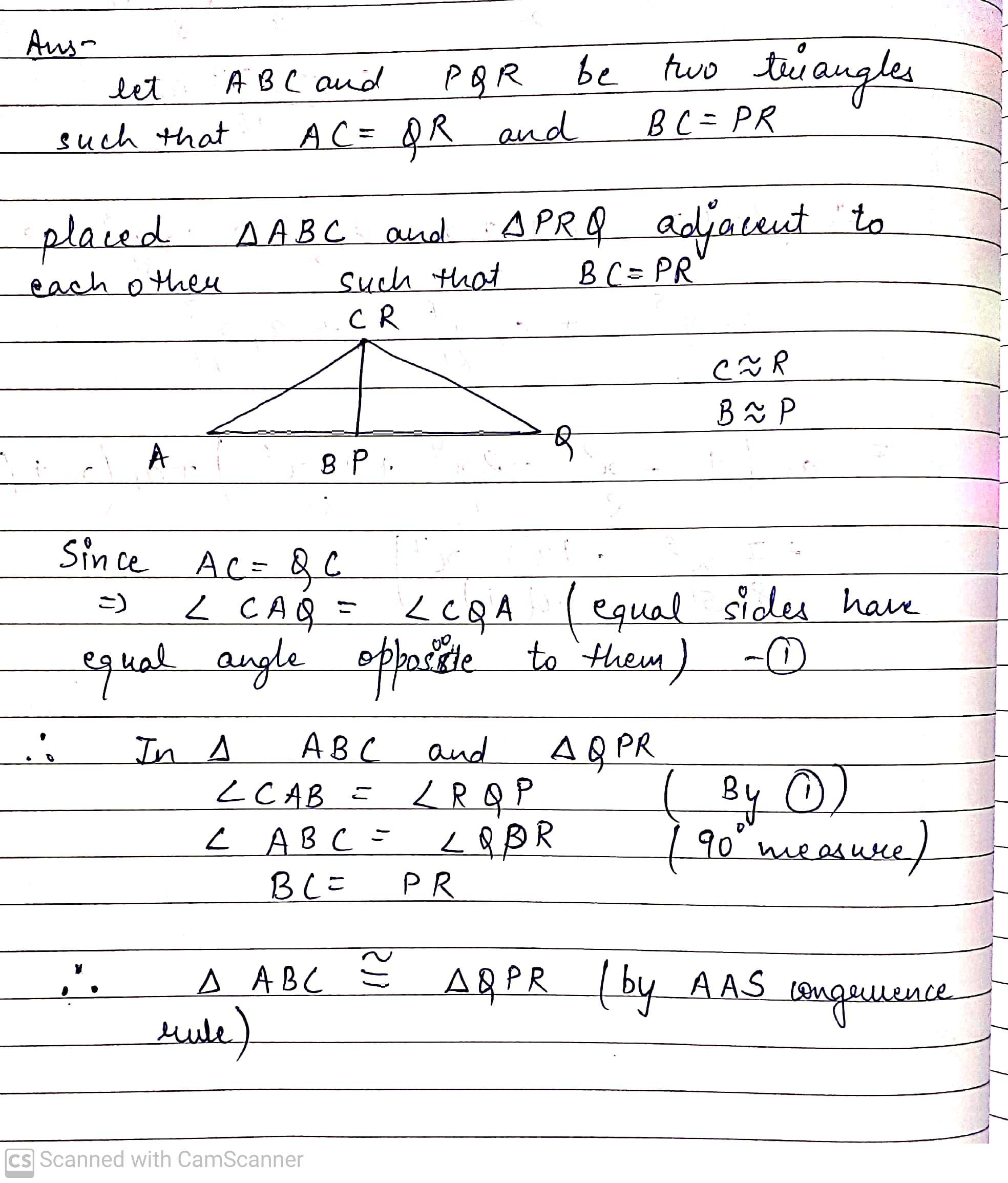#### Earn Coins

Coins can be redeemed for fabulous gifts.

Similar Homework Help Questions
• ### The Pythagorean Theorem for right triangles states that if a and b are the lengths of the legs of a right triangle and c is the length of the hypotenuse, then a2 C b2 D c2

The Pythagorean Theorem for right triangles states that if a and b are thelengths of the legs of a right triangle and c is the length of the hypotenuse,then a2 C b2 D c2

• ### Which theorem or postulate states that if the hypotenuse and an acute angle of one right triangle are congruent to the hypotenuse and corresponding acute angle of another right triangle, then the triangles are congruent

Which theorem or postulate states that if the hypotenuse and an acute angle of one right triangle are congruent to the hypotenuse and corresponding acute angle of another right triangle, then the triangles are congruent?

• ### the Pythagorean theorem to find the length of the unknown side of a right triangle, where...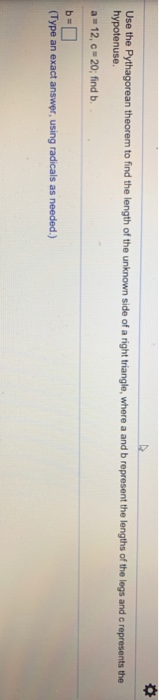the Pythagorean theorem to find the length of the unknown side of a right triangle, where a and b represent the lengths of the legs and c represents the hypotenuse. a 12, c 20, find b (Type an exact answer using radicals as needed.)

• ### Prove that if two right triangles have hypotenuses of equal length and an acute angle of...

Prove that if two right triangles have hypotenuses of equal length and an acute angle of one is equal to an acute angle of the other, then they are congruent.

• ### Can anyone help me prove the theorem 49 by using the following hint( Circle)? Here is...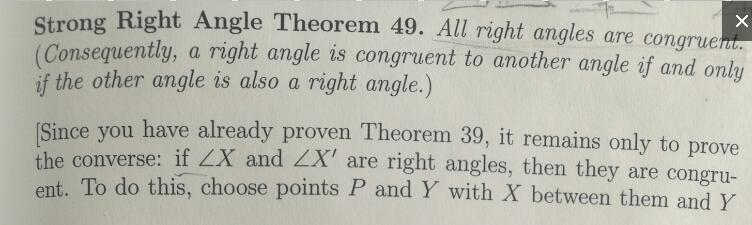Can anyone help me prove the theorem 49 by using the following hint( Circle)? Here is Theorem 39, can't use like right angles are 90 degrees, or that triangles are 180 degrees. Strong Right Angle Theorem 49. All right angles are congruer Consequently, a right angle is congruent to another angle if and only if the other angle is also a right angle.) Since you have already proven Theorem 39, it remains only to prove the converse: i f LX...

• ### 8.5 Theorem. Let s andt be any two different natural numbers with s t. Then (2st. (). is a Pythagorean triple. The prec...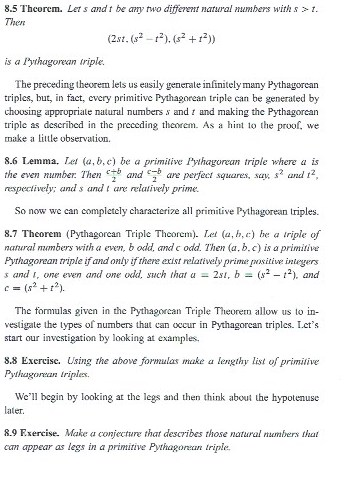8.5 Theorem. Let s andt be any two different natural numbers with s t. Then (2st. (). is a Pythagorean triple. The preceding theorem lets us easily generale infinitely many Pythagorean triples, but, in fact, cvery primitive Pythagorean triple can be generated by chousing appropriale natural numbers s and and making the Pythagorean triple as described in the preceding thcorem. As a hint to the proof, we make a little observation. 8.6 Lemma. Let (a, b,e) be a primitive Pythagorean...

• ### 2) (i) State the converse of the Alternate Interior Angle Theorem in Neutral Geometry. (ii) Prove...

2) (i) State the converse of the Alternate Interior Angle Theorem in Neutral Geometry. (ii) Prove that if the converse of the Alternate Interior Angle Theorem is true, then all triangles have zero defect. [Hint: For an arbitrary triangle, ABC, draw a line through C parallel to side AB. Justify why you can do this.] 5) Consider the following statements: I: If two triangles are congruent, then they have equal defect. II: If two triangles are similar, then they have...

• ### For this i presented problem number 80 from section 10.4. i need to answer the following question...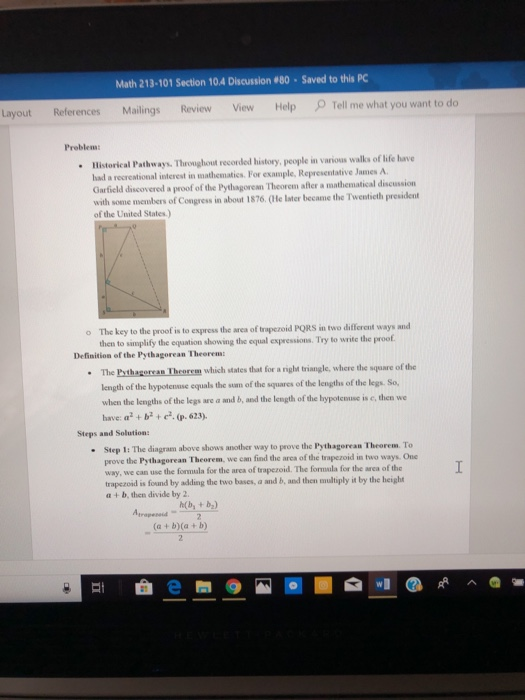for this i presented problem number 80 from section 10.4. i need to answer the following questions in the third picture. just those questions. no cursive please its hard to read. Math 213-101 Section 10.4 Discussion #80-Saved to this PC Layout References Mailings Review View Help Tell me what you want to do Problem: Historical Pathways. Throughout recorded history, people in various walls of life have had a recrentional interest in nathematics. For example, Represenlative James A Garfield discovered a...

• ### given isosceles triangle ABC, line AB = BC, and BD is the angle bisector of isosceles triangle ABC prove AB x DC = BC x AD Aren't the two halves congruent

given isosceles triangle ABC, line AB = BC, and BD is the angle bisector of isosceles triangle ABC prove AB x DC = BC x ADAren't the two halves congruent? (SAS) If so, then DC is equal to AD.Don't the ratios AB:AD=BC:DC by corresponding parts?how do i write that out like on a table though like step by stepI won't do the table otherwise that defeats the purpose of the assignment. If you do the exercise and post your work...

• ### PLEASE READ AND CODE IN BASIC C++ CODE. ALSO, PLEASE CODE USING THIS DO WHILE LOOP...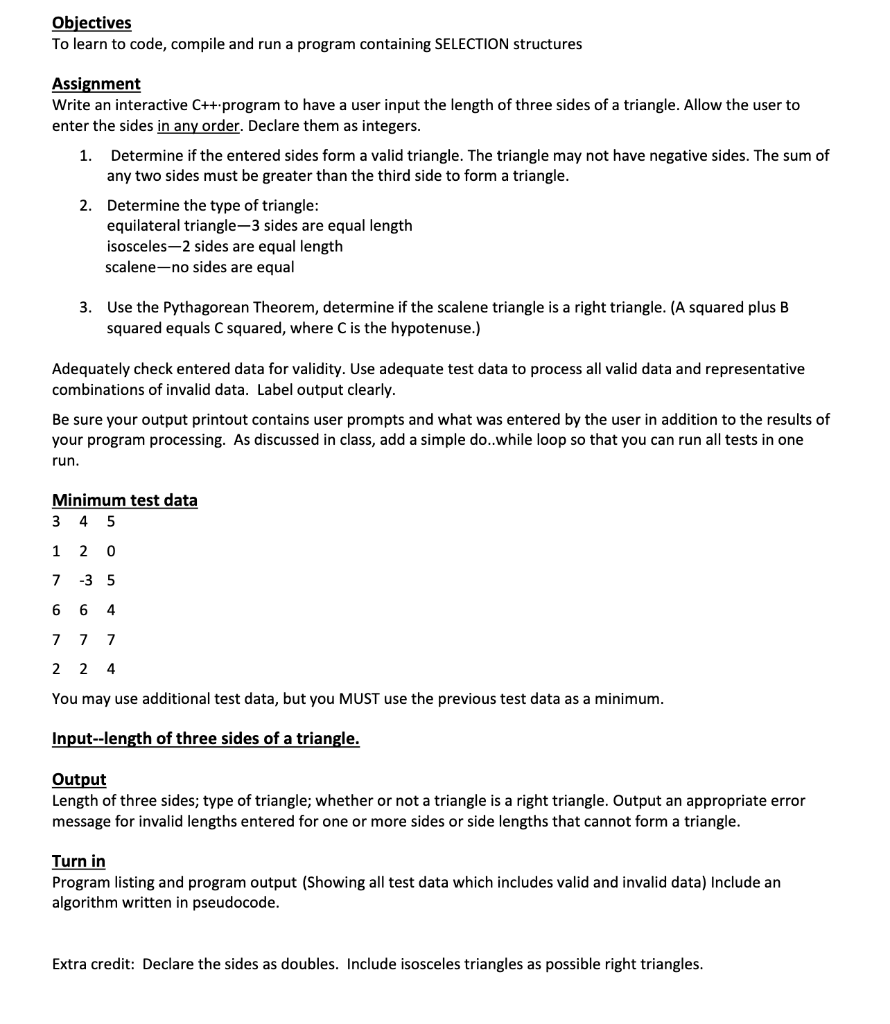PLEASE READ AND CODE IN BASIC C++ CODE. ALSO, PLEASE CODE USING THIS DO WHILE LOOP AND FORMAT: #include <iostream> using namespace std; int main() { //variables here    do { // program here             }while (true);    } Objectives To learn to code, compile and run a program containing SELECTION structures Assignment Write an interactive C++.program to have a user input the length of three sides of a triangle. Allow the user to enter the sides...